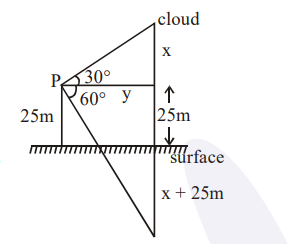# If the angle of elevation of a cloud from a pointQuestion:

If the angle of elevation of a cloud from a point $\mathrm{P}$ which is $25 \mathrm{~m}$ above a lake be $30^{\circ}$ and the angle of depression of reflection of the cloud in the lake from $P$ be $60^{\circ}$, then the height of the cloud (in meters) from the surface of the lake is :

1. 42

2. 45

3. 50

4. 60

Correct Option: , 2

Solution:$\tan 30^{\circ}=\frac{x}{y} \Rightarrow y=\sqrt{3} x$ .............(i)

$\tan 60^{\circ}=\frac{25+x+25}{y}$

$\Rightarrow \sqrt{3} y=50+x$

$\Rightarrow 3 x=50+x$

$\Rightarrow x=25 \mathrm{~m}$

$\therefore$ Height of cloud from surface $=25+25=50 \mathrm{~m}$